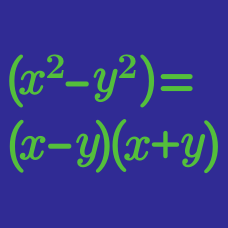Algebra

# Descartes Rule of Signs

Determine the number of sign changes in

$M(x) = x^6 + x^5 + x - 2.$

$T(x) = x^4 + x^3 - x^2 - 1$

By determining the types sign changes in $T(x)$ and $T(-x)$, determine the number of non-real complex roots of $T(x)$.

Given that the equation $x^9 - x^7 + 4 x^4 + x^2+100 = 0$ has either 1, 5, or 9 real roots, determine the number of its positive roots.

Let $J(x) = x^5 - 4x^4 + x^3 + 2x^2 + 3$. By comparing the values of $J(1)$ and $J(2)$, find the number of real roots of $J(x) = 0$.

Is there any negative root to the polynomial

$U(x) = x^7 + x- 7 ?$

×